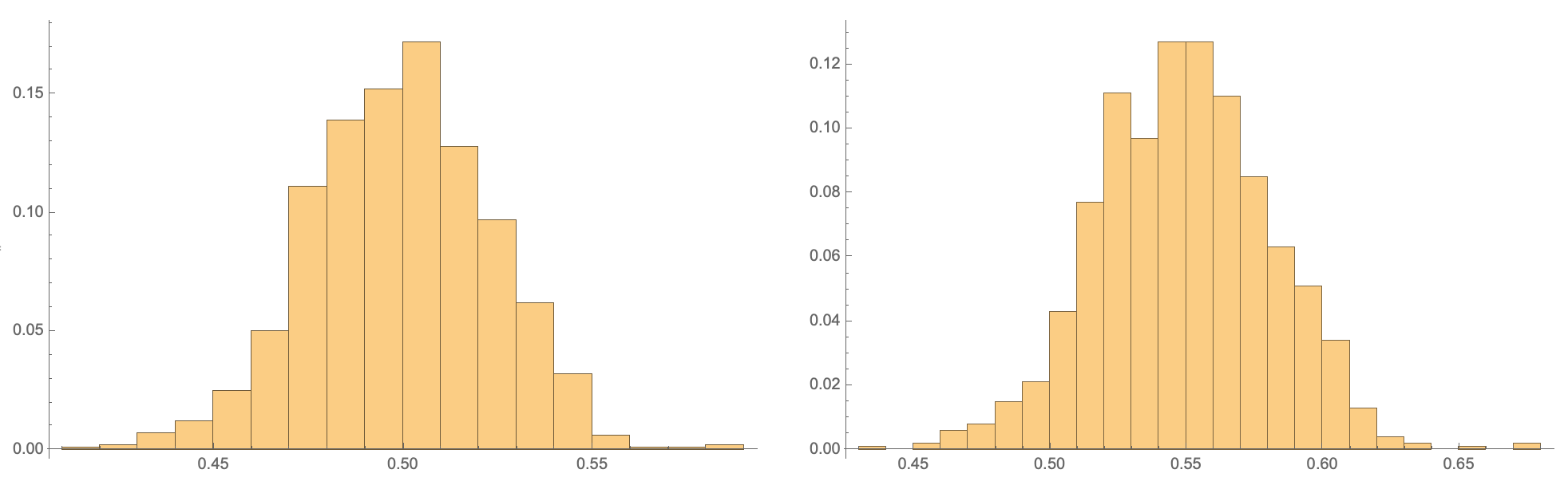# aliquote.org

We often make use of variable transformation to bring back the distribution of an explanatory variable to a more symmetric, gaussian-shaped, one. For instance, a log-transformation of a heavily skewed distribution (e.g., counts or doses with detect limit — which could otherwise be modeled as truncated or left-censored data) often helps in making the distribution less skewed, hence stabilizing the variance. As far as variance stabilization is concerned, there are other useful tricks one may not be necessarily aware.

Let’s consider the sample (Pearson or product-moment) correlation coefficient, $r$, which is computed as the ratio between the covariance and the product of the standard deviation of a bivariate sample $(X_1, Y_1), \dots, (X_n, Y_n)$. Using the delta method,1 it can be shown that $\sqrt{n}(r - \rho)$ is asymptotically zero-mean normal, with variance depending on a mix of the 3rd and 4th moment of $(X,Y)$, assumed to be bivariate normal. If the 4th moment exists, this is true for any underlying distribution. It can further be shown that:

$$\sqrt{n}(r - \rho) \rightarrow N\left(0, (1-\rho^2)^2\right).$$

This expression is not very handy in practice. But, first, how did we get there? Recall that the asymptotic confidence intervals (CI) for a parameter $\theta$ (here, the population correlation $\rho$) are asymptotically of level $1-2\alpha$ for every $\theta$ iff the asymptotic variance $\sigma^2(\theta)$ are independent of $\theta$. When it is not the case, we can apply the same idea to $\eta = \Phi(\theta)$, where the natural estimator of $\eta$ is $\Phi(T_n)$. If $\Phi$ is differentiable, then $\sqrt(n)\left(\Phi(T_n)-\Phi(\theta)\right) \rightarrow_\theta N\left( 0, \Phi'(\theta)^2\sigma^2(\theta)\right)$.2 If we choose $\Phi$ such that $\Phi'(\theta)\sigma(\theta)\equiv 1$, then the asymptotic variance is constant and finding an asymptotic CI for $\eta=\Phi(\theta)$ becomes easier. Indeed, $\Phi(\theta) = \int\frac{1}{\sigma(\theta)}d\theta$ is a variance-stabilizing (monotone) transformation, so that once we get a CI for $\eta$ we can easily build a CI for $\theta$.

In the case of the product-moment correlation, the variance-stabilizing transformation is:

$$\Phi(\rho) = \int \frac{1}{1-\rho^2}d\rho = \frac{1}{2}\log\frac{1+\rho}{1-\rho} = \text{arctanh}\:\rho.$$

In other words, $\sqrt{n}(\text{arctanh}\:r - \text{arctanh}\:\rho)$ converges to a standard Normal distribution for every $\rho$. Whence the following asymptotic CI for $\rho$:

$$\left( \text{tanh}\big(\text{arctanh}\:r-z_\alpha/\sqrt(n)\big), \text{tanh}\big(\text{arctanh}\:r+z_\alpha/\sqrt(n)\big)\right).$$

Here is a little simulation using 1000 replicates and theoretical correlation $\rho = 0.5$, with data displayed in the figure below (raw and transformed Pearson correlation in the left and right panel, respectively):

In:= data = Table[
Correlation[RandomVariate[BinormalDistribution[0.5], 10^3]][[1,
2]], {10^3}];
In:= GraphicsGrid[{{Histogram[data, Automatic, "Probability"],
Histogram[Map[ArcTanh, data], Automatic, "Probability"]}}]Figure 3.1 in van der Vaart2 is probably wrong since values for $\text{arctanh}\:r$ are all negative (right panel).

Unlike Box-Cox power transformation, where the idea is to look for a scale on which effects are additive,3 in this approach we directly work at the level of the test statistic. As an alternative, when the distribution of the test statistic is unknown or not close to normal, one can resort on computing bias-corrected bootstrap CIs.

1. Based on a Taylor expansion to approximate a random vector $\Phi(T_n)$ by the polynomial $\Phi(\theta) + \Phi'(\theta)(T_n-\theta)+\dots$ in $T_n-\theta$, the delta method is generally used to find the limit law of $\Phi(T_n)-\Phi(\theta)$ from that of $T_n - \theta$. ↩︎

2. van der Vaart, A.W. Asymptotic Statistics. Cambridge University Press, 1998. ↩︎

3. Box, G. E. P. and Cox, D. R. An analysis of transformations. Journal of the Royal Statistical Society B, 1964 (26): 211-252. ↩︎